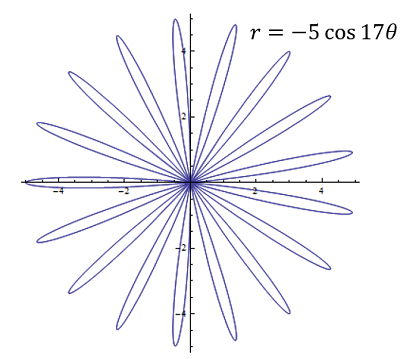# Use symmetry to find the exact area of the curve {eq}r = -5 \cos(17 \theta). {/eq}

## Question:

Use symmetry to find the exact area of the curve {eq}r = -5 \cos(17 \theta). {/eq}

## Rose Curve

Rose curves are curves obtained from graphing the polar equation,

{eq}r=a \cos k \theta {/eq},

where {eq}k {/eq} is some integer number defining the number of petals of the rose. Meanwhile the factor {eq}a {/eq} characterizes the size of the petals. For {eq}k=1 {/eq} the equation reduces to that of a circle (rose with one petal) with radius {eq}a {/eq}.

The polar equation,

{eq}r = -5 \cos(17 \theta). {/eq},

corresponds to a rose with 17 petals with equal areas as depicted in the diagram below.The area of one petal is obtained from the integral,

{eq}A=2 \displaystyle \int_0^{\pi/34} d\theta \int_0^{-5 \cos(17 \theta)} r \, dr {/eq}.

The factor of two is employed to take into account the symmetry inside each petal. The integration limits go from the middle point of the petal up to when it closes. Performing the integral,

{eq}\begin{align} A &=2 \displaystyle \int_0^{\pi/34} d\theta \int_0^{-5 \cos(17 \theta)} r \, dr \\ &=2 \int_0^{\pi/34} d\theta \dfrac{r^2}{2} \bigg|_0^{-5 \cos(17 \theta)} \\ &= \int_0^{\pi/34} d\theta 25 \cos^2 (17 \theta) \\ &=25\int_0^{\pi/34} \cos^2 (17 \theta) d\theta \\ &=25 \int_0^{\pi/34} \dfrac{1+2\cos (34\theta)}{2} \qquad \text{we used the double cosine identity}\\ &=\dfrac{25}{2} \int_0^{\pi/34} d\theta+ 25 \int_0^{\pi/34} \cos (34\theta) d\theta \\ &=\dfrac{25}{2} \dfrac{\pi}{34}+\dfrac{25}{34} \sin (34 \theta) \bigg|_0^{\pi/34} \\ &=\dfrac{25\pi}{68}. \end{align} {/eq}

Finally, the total area of the shape is 17 times this value,

{eq}A_T=17 \cdot \dfrac{25\pi}{68}=\mathbf{\dfrac{25\pi}{4}} {/eq}.Graphing Functions in Polar Coordinates: Process & Examples

from

Chapter 24 / Lesson 1
4.5K

Learn how to graph polar equations and plot polar coordinates. See examples of graphing polar equations. Transform polar to rectangular coordinates and vice versa.